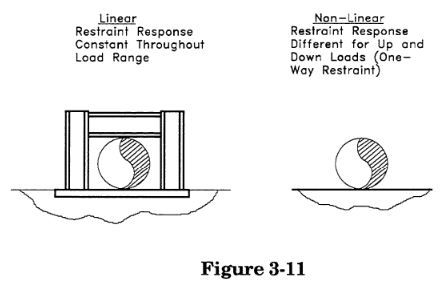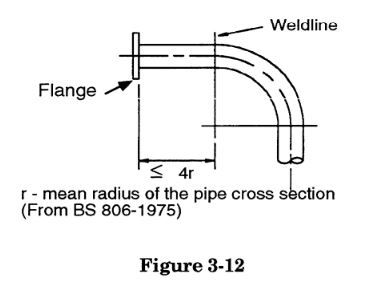top of page
Search

# 3.1 Computer Representation Of Basic Elements in CAESAR II

Pipe stress analysis computer software algorithms are based upon certain assumptions. These assumptions serve to make the computer model (and its corresponding analytical results) only an approximation of reality. In many cases this approximation may be sufficiently close to reality to fall within the tolerances, margins, and factors of safety of the problem to be an adequate representation, while in other cases the user may find it necessary to refine the model through more detailed modeling. This section describes the assumptions used in the computer algorithms in order that the user may more fully understand the limitations (and the potential work-arounds) of the system. The "stiffness method" algorithm, which is used to perform the actual analysis done by CAESAR II and other prominent pipe stress/structural computer programs is described in detail in Section 6 of these Pipe Stress Analysis Notes.Piping basic elements are modeled as centerline, or "stick" members. These elements are defined by two node points (one at the "from" end, and the other at the "to" end), each with fixed spatial coordinates and six degrees of freedom (three translational and three rota-tional).The elements are further defined by a constant (non-varying along the element length) set of stiffness parameters (i.e., material and cross-sectional properties). Response of the elements under load is governed according to recognized strength of material relationships (as described in Section 6 of these notes), subject to certain limiting assumptions. These assumptions, described on the following pages, govern the relationship between the mathematical model in the computer and the actual pipe existing in the power plant or refinery.

1 - All elements remain stable under load (local buckling of cross-sections is ignored):2 - Plane sections remain plane:The computer algorithm assumes that points A and B (of Figure 3-3) always lie on the same cross-sectional plane, whether in the deformed or the undeformed state.In reality, the moment F x L (in Figure 3-4) does not produce a uniform "plane-sections-remain-plane"bending load at the cross-section A-B, since it causes local warping.

3 - Hooke's Law is applicable across all fibers of the cross-section:4 - Hooke's Law is applicable throughout the entire load range:5 - Moments and forces applied to the beam are assumed to act about the neutral axis:6 - Element cross-sections do not ovalize under load (except as adjusted for bend elements):The stresses at the ovalized section are intensified due to:

1 - reduction in section modulus, and

2 - added local plate bending in the top and bottom fibers.

7 - Applied loads are not affected by the deformed state of the structure (P-delta effect)In reality, there will be an additional moment applied to the system, equal to the load times its displaced distance from the neutral axis of the structure (i.e., 1000 pounds x 0.25 inches = 250 in-lb). The computer software models this load as strictly a force with no applied moment.

8 - Rotational deformations of the system are assumed to be small:Node point rotations are added vectorially by the computer software. This is not a valid representation of reality for large rotations, as demonstrated in Figure 3-10 for three 90° rotations.

9 - Boundary conditions are assumed to respond in a linear fashion:The stiffness algorithm cannot solve for non-linear restraint conditions, such as one-directional restraints, bi-linear restraints (soil or bottomed out springs), friction, etc. However, CAESAR II does include a procedure which overcomes this limitation; see point 8 below.

These limitations are of the most concern when modeling the following situations (pointers for increasing accuracy in each situation are also given):

• Large Diameter/thin wall piping or ducts: In this case, it is advisable to minimize localized loadings by distributing them with pads or saddles, or do plate buckling analysis (preferably with finite element software) when the loads cannot be altered.

• Localized stress conditions not explicitly covered by an SIF, i.e. a saddle: The portion of the pipe impacted by the saddle may be modeled as a rigid element, while saddle/piping local stresses may be estimated through the use of finite element analysis or through the use of Welding Research Council Bulletins, such as 107 and 198.

• Pipe connections to thin walled vessels: The flexibility of the connection may be modeled by a flexible element (such as that generated using Welding Research Council Bulletin 297), while stresses in the pipe and vessel may be estimated through the use of finite element analysis or through the use of Welding Research Council Bulletins 107 and 297.

• Highly corrosive systems (especially when subjected to cyclic loadings): Corrosion of a pipe results in an irregular cross-section which is usually modeled by using the uncorroded cross-section for load generation (weight and thermal forces), and the fully corroded cross- section for calculation of the section modulus (stress calculation). Corrosion is much more dangerous under fatigue loadings due to the fact that it provides many more opportunities for crack initiation; in order to compensate, a low cyclic reduction factor should be used to match the allowable expansion stress range to the fatigue curve for a highly corroded material.

• Elbows: Elbows ovalize significantly when subjected to bending loads. This can be accounted for by increasing the flexibility of the elbow element in the computer model and multiplying the calculated stress by a stress intensification factor (this is done automatically by most programs such as CAESAR II). Code defined "flexibility factors" for bends have been determined theoretically and verified experimentally.The flexibility and stress intensification factors of bends must be reviewed in those cases where ovalization is inhibited (such as when the elbow is stiffened by flanges or welded attachments). The piping codes provide correction factors for bends with one or two flanges, but omit geometries such as shown in Figure 3-13.These attachments almost certainly affect the flexibility, and more importantly, the stress intensification factors for the bends. The factors for heavily stiffened bends, such as that shown in Figure 3-13(A), could be estimated using finite element analysis, or stiffness could be increased by modeling the elbows as flanged, or simply as straight pieces of pipe (with increased stress intensification factors applied). In less pronounced cases such as those shown in Figures 3-13 (B) and (C), deviations from the response of an unstiffened bend is usually ignored.

• Loadings which produce stresses which are well outside of the code allowable ranges: These loads will tend to produce stresses well beyond the material yield stress, stresses in the buckling range, large displacements resulting in significant P-delta loads, or large rotations (leading to inaccurate results). This limits programs such as CAESAR II as accurate analysis tools throughout the full range of potential loadings. However accuracy is not affected for those loads which are of most interest to the engineer—code allowables are based upon the fact that the analysis being done assumes linear material response.

• Non-linear boundary conditions: The effects of non-linear restraints must be simulated through an iterative process aimed at convergence of the non-linear restraints in legitimate states—for example, with the pipe lifted off at a one-way support (and with the support function removed from the analysis), or with the pipe sliding along a frictional restraint (and with an appropriate force applied along that line of action in the analysis). This process is activated (during static analysis) automatically when a non-linear effect is detected by CAESAR II.

• Non-homogenous elements: As noted, piping elements are modeled as stick elements of constant cross-section and material properties. In certain cases, such as with reducers, which have a variable cross-section, this is not a valid representation. An element such as this is usually modeled as a single, or as a series of elements, each having average parameters. For example, a 12x8 standard wall reducer may be modeled as a 10-inch standard wall pipe (approximately the average of the inlet and outlet pipes), or as two segments, with outer diameters and wall thicknesses interpolated between the two. When using those codes which define a stress intensification factor for reducers, one would have to be calculated and specifically applied at that location.

• Rigid elements: Rigid elements, such as valves and flanges are most difficult to model due to the inability to represent their geometry, and their stress distribution with stick elements. Therefore, pipe stress software cannot be used to accurately determine the effects of the piping system on rigid elements. Analysis of these components is best left to finite element analysis, test, or other recognized methods. However, the effects of the rigid elements on the piping system can be simulated by providing an element of high relative stiffness in the model (it is always more important to adequately model relative stiffnesses than absolute stiffnesses when constructing a model). This is done by providing an element with sufficiently large cross-section, and having the defined weight of the rigid item. In CAESAR II, a rigid element is modeled as having:

a) an insulation factor of 1.75 (compared to the matching pipe), unless a zero weight rigid (a modeling construct) b) fluid weight of the matching pipe added, unless a zero weight rigid c) the same inside diameter and 10 times the wall thickness of the matching pipe